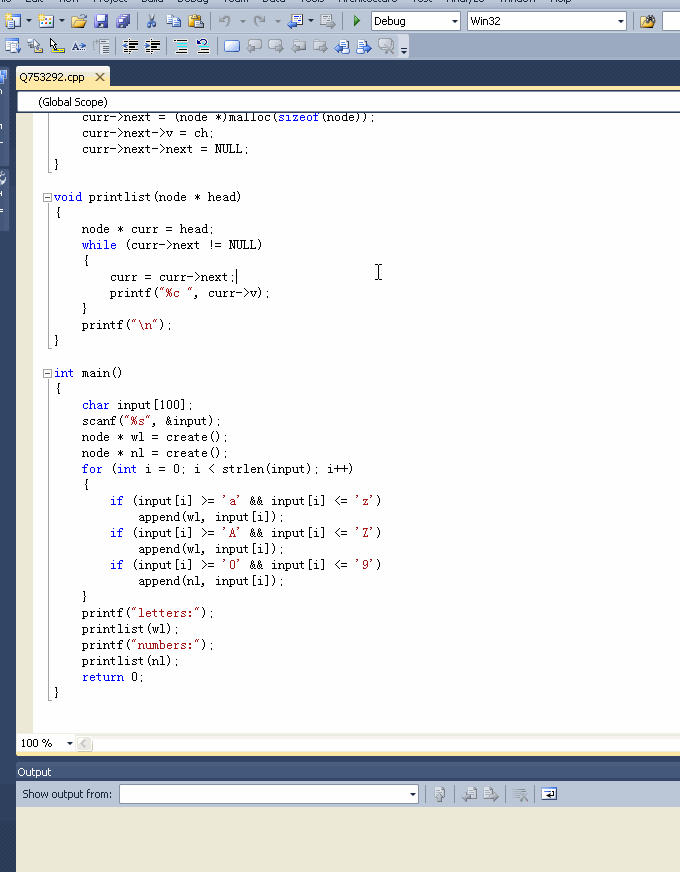C语言求助：输入一个字符串,将其中的字母字符输入一个链表,将其中的数字字符输入另一个链表。

1个回答

# 如果问题得到解决，请点我回答做上角的采纳，采纳后可以继续回答你的别的问题

``````// Q753292.cpp : Defines the entry point for the console application.
//

#include "stdafx.h"

#include "stdio.h"
#include "stdlib.h"
#include "string.h"

struct node
{
char v;
node * next;
};

node * create()
{
node * r = (node *)malloc(sizeof(node));
r->v = '\0';
r->next = NULL;
return r;
}

void append(node * head, char ch)
{
while (curr->next != NULL) curr = curr->next;
curr->next = (node *)malloc(sizeof(node));
curr->next->v = ch;
curr->next->next = NULL;
}

{
while (curr->next != NULL)
{
curr = curr->next;
printf("%c ", curr->v);
}
printf("\n");
}

int main()
{
char input;
scanf("%s", &input);
node * wl = create();
node * nl = create();
for (int i = 0; i < strlen(input); i++)
{
if (input[i] >= 'a' && input[i] <= 'z')
append(wl, input[i]);
if (input[i] >= 'A' && input[i] <= 'Z')
append(wl, input[i]);
if (input[i] >= '0' && input[i] <= '9')
append(nl, input[i]);
}
printf("letters:");
printlist(wl);
printf("numbers:");
printlist(nl);
return 0;
}

``````